# Constructing Triangles

Constructing triangles will include the construction of different triangles using a protractor, a compass and a ruler. A triangle is a three-sided polygon. It has three sides, three vertices and three angles. Construction of triangles is easy when the measurements are given to us based on different properties such as SSS, SAS and ASA.

Let us learn here to construct a triangle with given measurements. Also, learn to draw triangles of some special cases.

## How to Construct Triangles?

To construct a triangle one should know these properties and rules:

• All three sides are given (SSS – Side side side)
• Two sides and included angle are given (SAS – Side angle side)
• Two angles and the included side is given (ASA – Angle side angle)
• The measure of the hypotenuse and a side is given in the right triangle (RHS – Right angle hypotenuse side)

For constructing triangles from given data, we generally make use of the given congruency conditions and construct the required triangle.

### Constructing triangle With SSS Property

When the length of three sides of the triangle is given, then follow the below steps to construct the required triangle.

• Draw a line segment AB, of length equal to the longest side of the triangle
• Now using a compass and ruler take the measure of the second side and draw an arc
• Again take the measure of the third side and cut the previous arc at a point C
• Now join the endpoints of the line segment to point C and get the required triangle ABC

### Constructing triangle with SAS Property

When the length of two sides and the angle included between them are given, then use the following steps to construct the triangle.

• Draw a line segment AB, of length equal to the longest side of the triangle, using a ruler
• Put the center of the protractor on one end of a line segment (say A) and measure the given angle. Join the points and construct a ray, such that the ray is nearer to the line segment AB
• Take the measure of another given side of the triangle using a compass and a ruler
• Put the compass at point A and cut the ray at another point, C
• Now join the other end of the line segment, i.e., B to the point C
• Hence, the triangle ABC is constructed

### Constructing triangle with ASA Property

When the measures of two angles and the side included between them are given of a triangle, then it is said to be ASA congruency. Follow the given steps to draw a triangle with ASA property.

• Draw a line segment AB, of length equal to the given side of the triangle, using a ruler
• At one endpoint of line segment (say A) measure one of the given angles and draw a ray AR
• At another endpoint of line segment (i.e.,B) measure the other angle using a protractor and draw the ray BQ, such that it cuts the previous ray at a point P
• Join the previous point P, with both the endpoints A and B of the line segment AB, to get the required triangle

### Construction triangle with RHS Property

When the hypotenuse side and any one of the other two sides of right triangle are given, then it is RHS property. Follow the given steps to draw a triangle with RHS property.

• Draw the line segment AB, equal to the measure of hypotenuse side
• At one endpoint, say A, of line-segment measure the angle equal to 90 degrees and draw a ray, AR
• Measure the length of another given side and draw an arc to cut the ray AR at a point P
• Now join the point P and B to get the required right triangle

## Special Cases of Constructing Triangles

There are few special cases of constructing triangles that are discussed here.

### Construction of Triangles – Case 1

Given the base of a triangle, its base angle and the sum of the other two sides

For constructing ∆ABC such that base BC, base angle ∠B and the sum of other two sides, i.e. AB + AC are given, the following steps of construction is followed:

Steps of Construction of a Triangle

Step 1. Draw the base BC of ∆ABC as given and construct ∠XBC of the given measure at B as shown.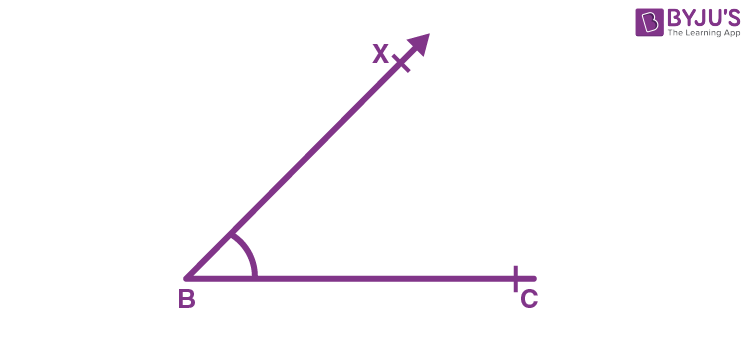Step 2. Keeping the compass at point B cut an arc from the ray BX such that its length equals AB + AC at point P and join it to C as shown in the figure.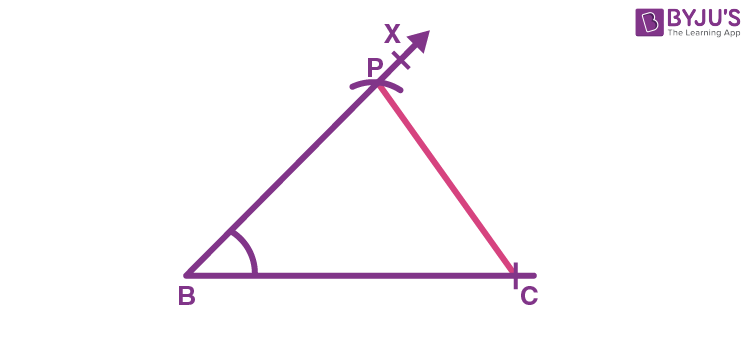Step 3. Now measure ∠BPC and from C, draw an angle equal to ∠BPC as shown in the figure.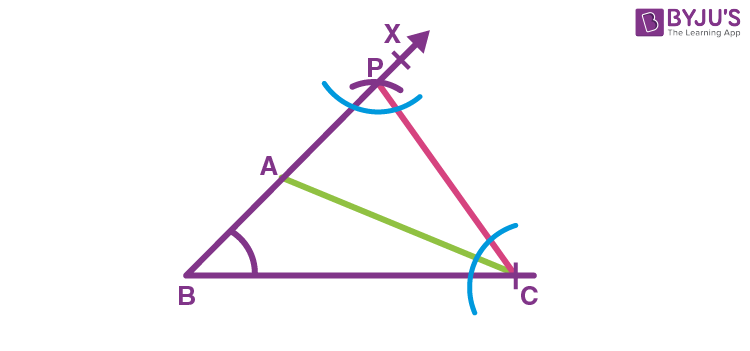∆ABC is the required triangle. This can be proved as follows:

 Sl.No Statement Reason Base BC and ∠B are drawn as given. Now in ∆ACP, 1 ∠ACP = ∠APC By Construction 2 AC = AP ∆ACP is isosceles 3 AB = BP – AP = BP – AC From Statement 2 4 AB + AC = BP Proved

### Construction of Triangles – Case 2

Given the base of a triangle, its base angle and the difference between the other two sides

For constructing ∆ABC such that base BC, base angle∠B and difference of the other two sides, i.e. AB – AC or  AC-AB is given, then for constructing triangles such as these two cases can arise:

1. AB > AC
2. AC > AB

The following steps of construction are followed for the two cases:

Steps of Construction if AB > AC:

Step 1. Draw the base BC of ∆ABC as given and construct ∠XBC of the required measure at B as shown.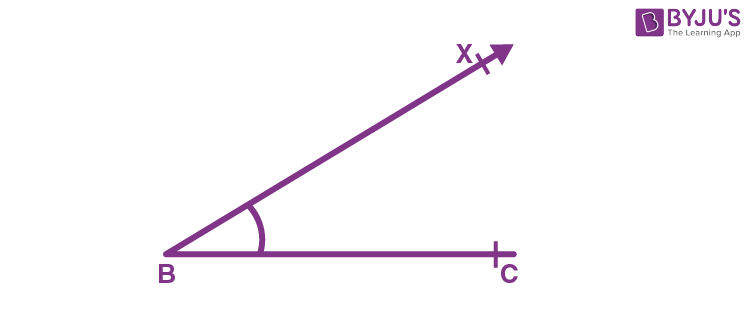Step 2. From the ray, BX cut an arc equal to AB – AC at point P and join it to C as shown

Step 3. Draw the perpendicular bisector of PC and let it intersect BX at point A as shown: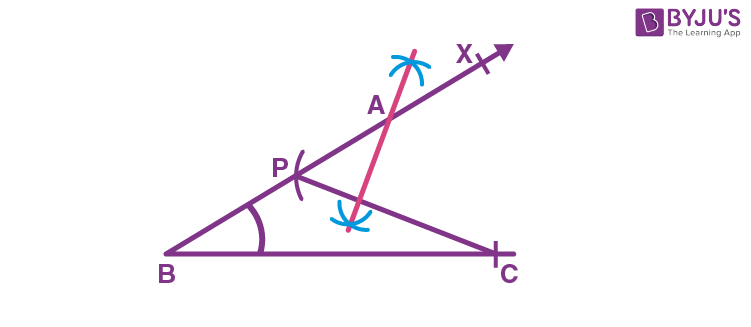Step 4. Join AC, ∆ABC is the required triangle.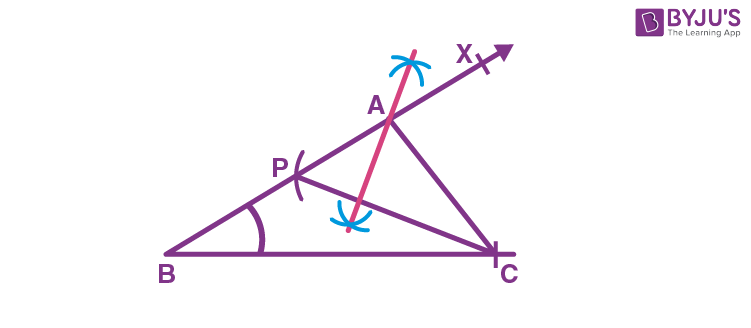Steps of Construction if AC > AB:

Step 1. Draw the base BC of ∆ABC as given and construct ∠XBC of the required measure at B as shown.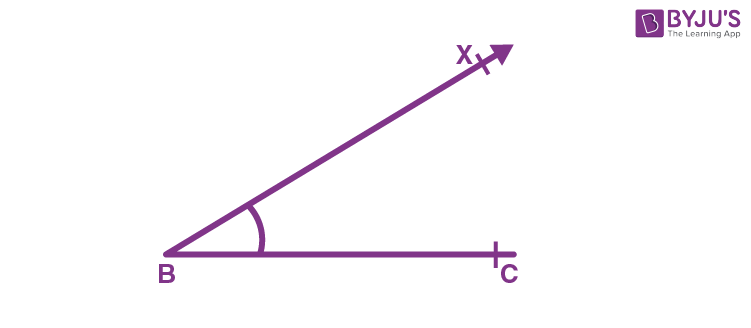Step 2. On the ray BX cut an arc equal to AB – AC at point P and join it to C. In this case, P will lie on the opposite side to the ray BX. Draw the perpendicular bisector of PC and let it intersect BX at point A as shown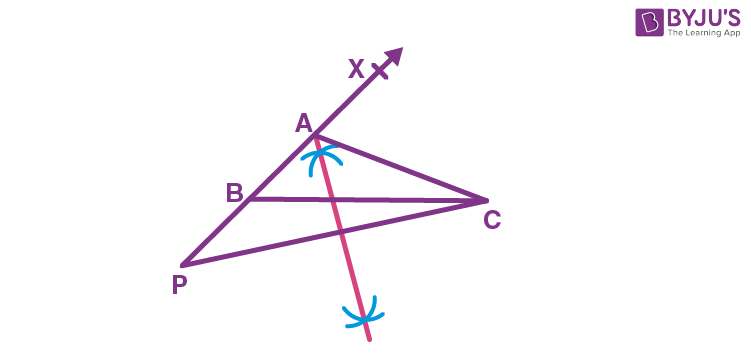Step 3. Join the points A and C, and hence ∆ABC is the required triangle.

### Video Lesson on Triangles## Practice Questions on Constructing Triangles

1. Construct a triangle with sides equal to 4 cm and 6 cm and the angle included between them is 40°.
2. Construct a triangle with two angles 40° and 70° and the side included between them is of length 8cm.
3. Construct a triangle with given three sides AB = 3cm, BC = 5cm and AC = 6cm.

## Frequently Asked Questions on Contructing triangles

Q1

### What are the rules of constructing triangles?

A triangle can be constructed based on four rules or properties:
All three sides are given (SSS – Side side side)
Two sides and included angle are given (SAS – Side angle side)
Two angles and the included side is given (ASA – Angle side angle)
The measure of the hypotenuse and a side is given in the right triangle (RHS – Right angle hypotenuse side)
Q2

### How to construct a triangle with given three sides?

Draw a line segment AB, of length equal to the longest side of the triangle
Now using a compass and ruler take the measure of the second side and draw an arc
Again take the measure of the third side and cut the previous arc at a point C
Now join the endpoints of the line segment to the point C and get the required triangle ABC
Q3

### How many kinds of triangles are there?

The six types of triangles are isosceles triangle, equilateral triangle, scalene triangle, obtuse triangle, acute triangle and right triangle.